Disclosure: This post may contain affiliate links, meaning when you click the links and make a purchase, we receive a commission.

# Using VLOOKUP with IF Condition in Excel (5 Examples)

VLOOKUP is one of the most powerful and top used functions in Excel. Using IF logical function with VLOOKUP makes the formulas more powerful.

In this article, we shall see a good number of examples where we have paired VLOOKUP with IF condition in Excel.

At first, let’s take a closer look at both the Excel IF and VLOOKUP functions. This will make it easy to understand the formulas.

## Excel IF Function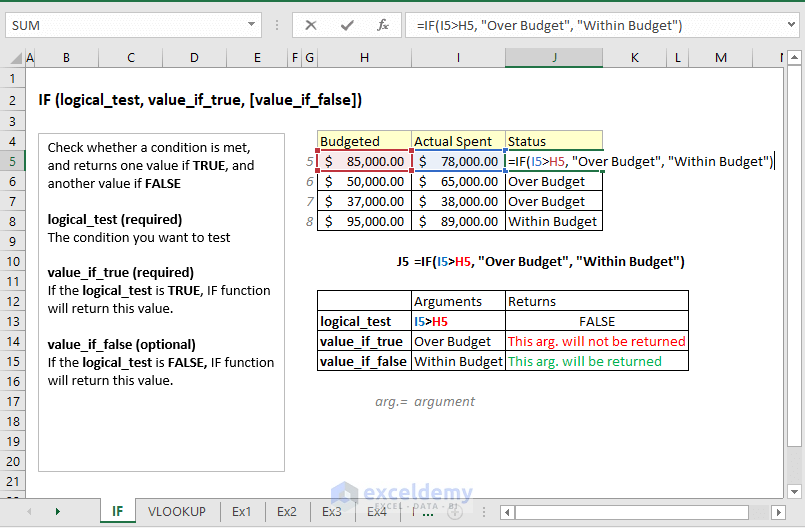## Excel VLOOKUP Function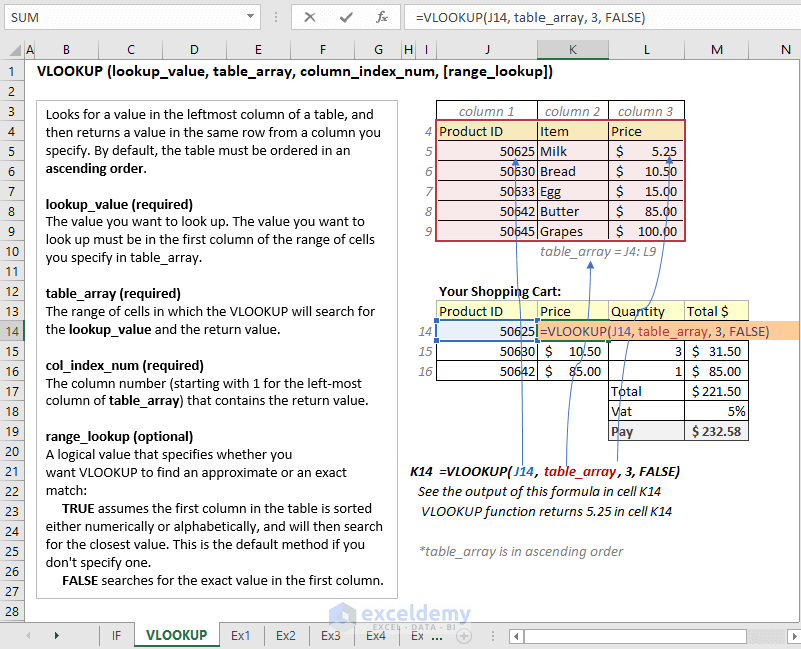## 1) Using VLOOKUP and IF condition to Choose the best Bargain Store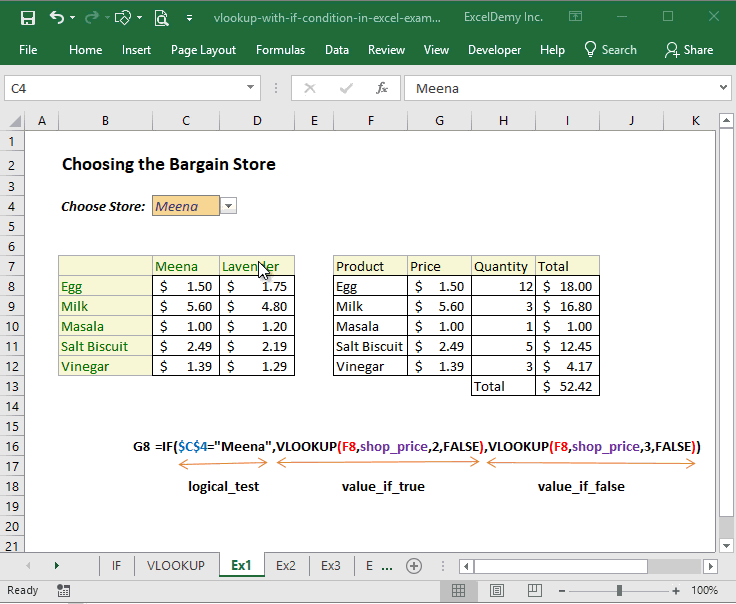### How does this formula work?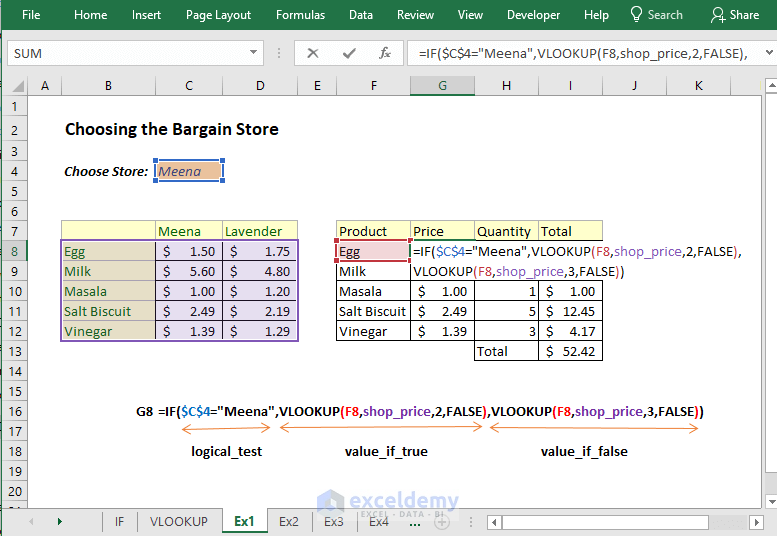This is a very simple formula. Let me explain the formula in cell `G8 = IF(\$C\$4="Meena",VLOOKUP(F8,shop_price,2,FALSE),VLOOKUP(F8,shop_price,3,FALSE))`

• shop_price = \$B\$8:\$D\$12
• IF Function tests whether \$C\$4 cell value is equal to value Meena.
• If the above logical test is TRUE, it returns this part of the formula VLOOKUP(F8,shop_price,2,FALSE). It is a straightforward VLOOKUP formula. It searches for the value of cell F8 in the shop_price table array and if it finds there then returns the value of the 2nd column of the same row.
• If the logical test is FALSE, then it returns this part of the formula VLOOKUP(F8,shop_price,3,FALSE). A simple VLOOKUP formula. VLOOKUP finds the value of the F8 cell in the shop_price table array and if it finds then returns the value of the 3rd column of the same row.

## 2) Excel VLOOKUP with 2 Tables of Values

In this example, you will see how to use two or more table arrays in Excel VLOOKUP formula.

In the following image, you’re seeing how I have made a formula using VLOOKUP and IF functions to choose one of the two table arrays.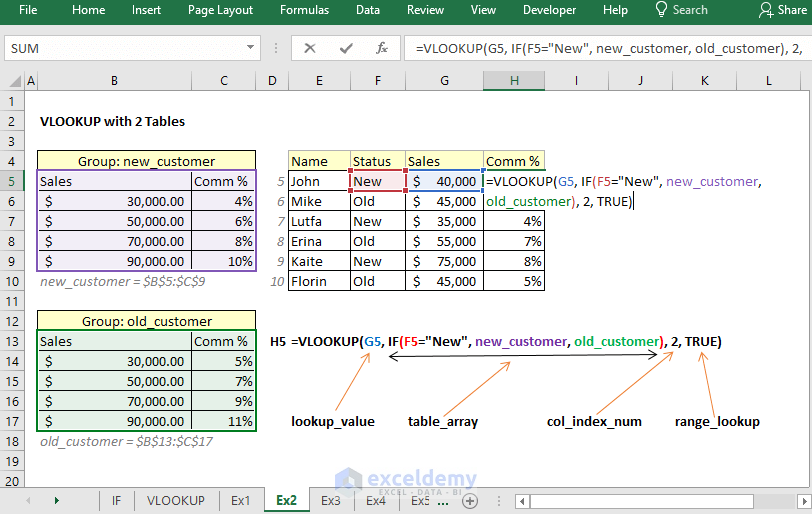### How does this formula work?

The image above is self-explanatory. Here is the explanation for the complete laymen:

• In cell H5, I have used this formula: `=VLOOKUP(G5, IF(F5="New", new_customer, old_customer), 2, TRUE)`
• G5 is the lookup_value here and it is an amount under the Sales column.
• `IF(F5="New", new_customer, old_customer)`: This formula will return one of the two tables: new_customer and old_customer. new_customer = \$B\$5:\$C\$9 and old_customer = \$B\$13:\$C\$17.
• The rest is simple. Column index number is 2. So, the VLOOKUP function will return value from the 2nd column of the same row where it finds the lookup value.
• I have used TRUE value as the range_lookup argument, so the VLOOKUP function will search for the closest value equal to or less than the lookup value.

## 3) Using VLOOKUP function as the logical test of IF function (good for inventory management)

This is a good example if you manage inventory using Excel. In the following worksheet (top left corner), you’re seeing I have a table. The table lists some products and their status under the Availability column.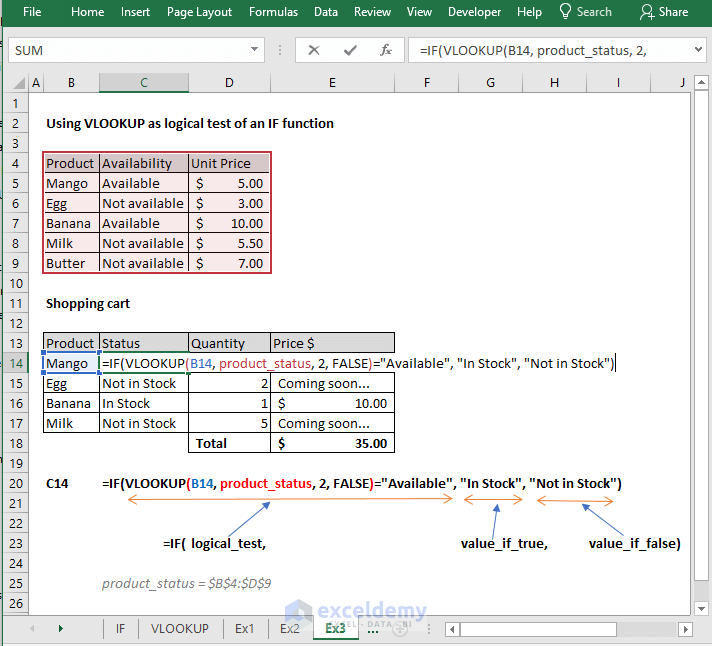In the 2nd table (under the Shopping Cart), under the Status column, and in cell C14 I have input this formula:

`=IF(VLOOKUP(B14, product_status, 2, FALSE)="Available", "In Stock", "Not in Stock")`

### How does this formula work?

This formula is also self-explanatory from the above image. Here is the explanation for new Excel users:

Let’s explain the logical_test argument of this formula. We are using this formula `VLOOKUP(B14, product_status, 2, FALSE)="Available"`as the IF function’s logical_test argument. If this part of the formula returns TRUE value then the cell will show “In Stock” value, otherwise it will show “Not in Stock” value.

Check out the image below.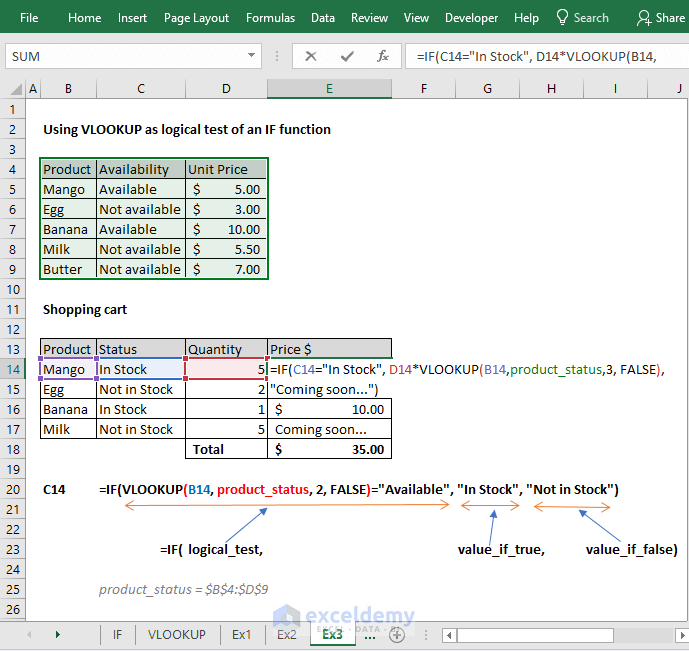We have also used another IF and VLOOKUP combo under the Price \$ column.

This is the formula I have used in cell E14 `=IF(C14="In Stock", D14*VLOOKUP(B14,product_status,3, FALSE), "Coming soon...")`

If the value of cell C14 is “In Stock”, then the cell will show the value of this part of the formula: D14*VLOOKUP(B14,product_status,3, FALSE). This formula is just a product of D14 cell value and a simple VLOOKUP formula.

If the value of cell C14 is not “In Stock”, then the cell will show this value “Coming soon…”.

## 4) Selecting col_index_num argument of VLOOKUP function dynamically with IF function

Look at the following image. You see when I select Projected, it is selecting the values under the Projected column of the first table (on the top left corner of the worksheet). When I am selecting the Actual Sales value, the table below is showing the values of Actual Sales column from the first table.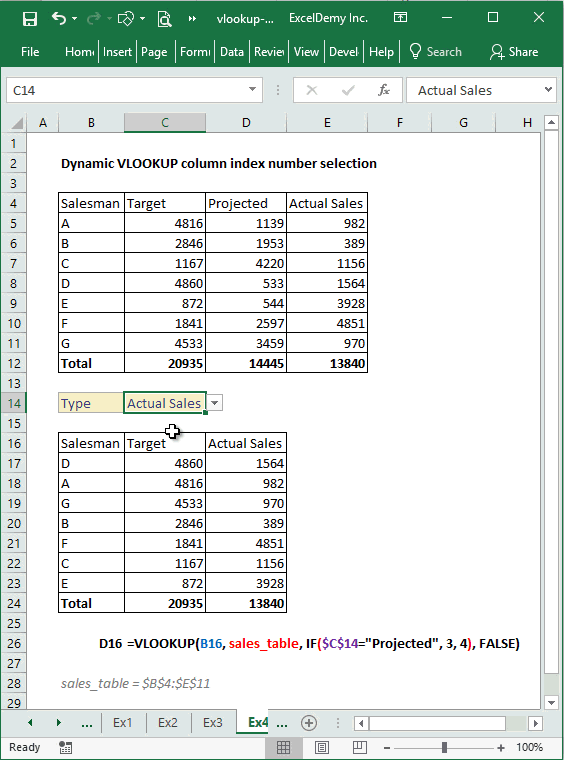This technique will help you a lot when you will make dashboards using Excel.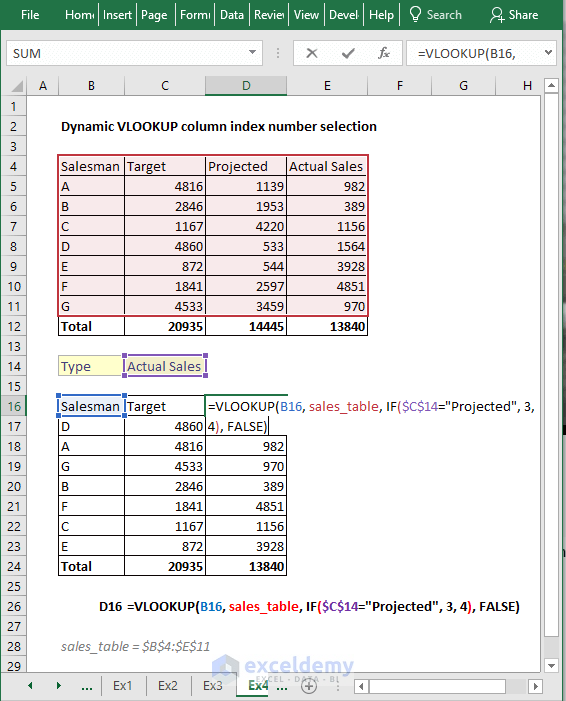In cell C14, I have made a data validation to show only two values: Projected and Actual Sales.

Then in cell D16, I have used this formula `=VLOOKUP(B16, sales_table, IF(\$C\$14="Projected", 3, 4), FALSE)`. Then dragged down this formula for other cells in the same column.

### How does this formula work?

This is a straightforward simple VLOOKUP formula. We have just made the col_index_num argument part dynamic using an IF function.

This part of the formula demands a little discussion: `IF(\$C\$14="Projected", 3, 4)`. If \$C\$14 value is equal to value Projected, the IF function will return 3, otherwise, it will return 4. So, this is dynamically selecting the column index number of VLOOKUP formula. Quite interesting!

## 5) VLOOKUP with IFERROR and IF + ISNA Functions

These two techniques will help you to handle #N/A error. VLOOKUP generates #N/A error when it does not find a value that you’re looking for.

Look at the following image intensively.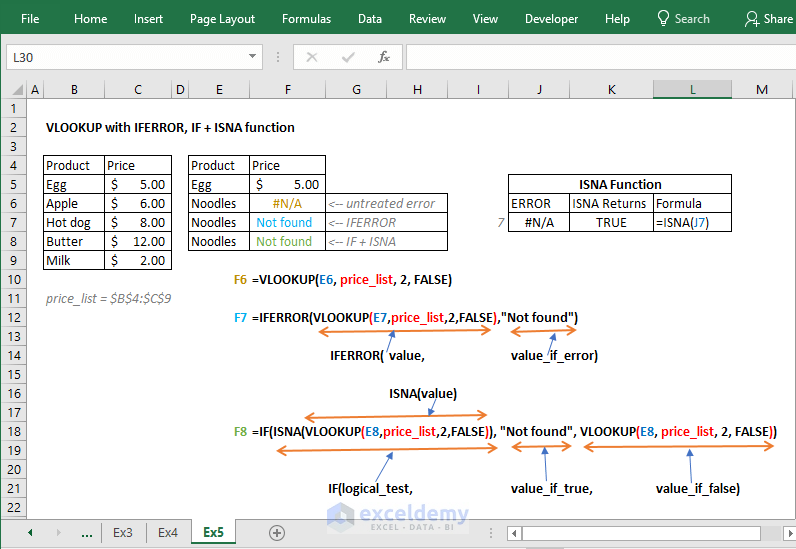Cell F6 shows the #N/A error as we did not handle the error smartly.

But cell F7 and F8 does not show any error though VLOOKUP did not find the Noodles product in the leftmost table (our table_array).

Let’s explain these two formulas:

### Cell F7 Formula

In cell F7, we have this formula: `=IFERROR(VLOOKUP(E7,price_list,2,FALSE),"Not found")`. As the value of IFERROR function, we have input the VLOOKUP formula. If this VLOOKUP formula returns an error, then “Not found” value will be shown in the F7 cell.

### Cell F8 Formula

Let’s explain the formula of cell F8. We have used this formula in cell F8: `=IF(ISNA(VLOOKUP(E8,price_list,2,FALSE)), "Not found", VLOOKUP(E8, price_list, 2, FALSE))`.

ISNA function returns TRUE when it finds the #N/A error. I have shown it on the top right corner of the worksheet.

As the ISNA function’s value, I have input the VLOOKUP formula: `VLOOKUP(E8,price_list,2`,FALSE`)`.

If this formula returns the #N/A error, then ISNA will return a TRUE value and IF function’s logical_test argument will be TRUE. If this VLOOKUP formula returns a real value, ISNA will return a FALSE value.

So, if ISNA returns the TRUE value, IF function’s this value “Not found” will be shown in the cell F8. Otherwise, this formula will be executed: `VLOOKUP(E8, price_list, 2, FALSE)`. This is a straightforward VLOOKUP formula.

So, these are my ways to use VLOOKUP with IF condition in Excel. I have shown total 5 examples.

Happy Excelling 😊

Hello!
Welcome to my Excel blog! It took me some time to be a fan of Excel. But now I am a die-hard fan of MS Excel. I learn new ways of doing things with Excel and share here. Not only how to guide on Excel, but you will get also topics on Finance, Statistics, Data Analysis, and BI. Stay tuned!

1. ReplyDaniel González Jun 24, 2018 at 11:33 PM

In the 4th way, I commonly use MATCH instead IF, because if I have more than two items, MATCH works very well

The col_index argument will be like this:
MATCH(\$C\$14,\$B\$4:\$E\$4,0)

By the way, great job!! Keep going!

Have a nice day!

• ReplyJun 25, 2018 at 11:55 AM

Hi Daniel,
Yes. INDEX and MATCH combo work in a more versatile way than the VLOOKUP function. I did not use Index Match because I wanted to show all the examples with VLOOKUP and IF Functions.
Keep in touch.
Best regards
Kawser

2. ReplyMar 7, 2019 at 11:25 AM

interesting function & I can’t dispense it <3

• ReplyMar 7, 2019 at 7:58 PM

3. ReplyFerreira Mar 7, 2019 at 6:10 PM

Congratulations, great!

• ReplyMar 7, 2019 at 7:58 PM

Thanks, Ferreira!

4. ReplySuryaHS Mar 8, 2019 at 8:15 AM

Thank you for the useful tutorial, it explained the uses very easily and effectively. 🙂

5. ReplyJoe Jul 17, 2019 at 10:08 PM

Nice code, but your explanations are rather condescending. “For the complete layman”…? Comes off as superior.

• ReplyJul 18, 2019 at 6:20 PM

Maybe a little bit tough for the complete layman. I shall change my Phrase then 🙂